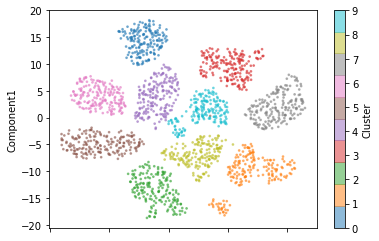# UMAP & MNIST¶

This notebook walks you through the use of a really amazing TSNE-like dimensionality reduction technique called UMAP on the MNIST dataset to terraform a Planetoid. Follow the link to learn more about it and get it installed.

In :
import planetoids as pt
import pandas as pd


Technically, you can use any labelled data in two dimensions to generate a new planetoid. However, this package was specifically designed with algorithms like UMAP in mind for dealing with larger datasets.

You will have to play around a bit with the hyperparameters of your chosen algorithm to produce some nice separated clusters.

I like the pairing of UMAP & Planetoids as it produces globular clusters with a convenient API to control the density of the produced clusters.

In :
from sklearn.datasets import load_digits
from umap import UMAP
import matplotlib.pyplot as plt

#reduce the data down to two dimensions using
#here we are specifically using UMAP in a supervised
#manner leveraging the target labels
reducer = UMAP(n_components=2,
min_dist=1.7,
target_weight=0.5,
random_state=42,
n_epochs=50
)

embedding = reducer.fit_transform(data.data, y=data.target)

reduced = pd.DataFrame(embedding, columns=['Component1', 'Component2'])
reduced['Cluster'] = data.target

reduced.plot(kind='scatter', x='Component2', y='Component1', c='Cluster', cmap='tab10', s=3, alpha=0.5)
plt.show()

C:\ProgramData\Anaconda3\lib\site-packages\umap\spectral.py:229: UserWarning:

Embedding a total of 4 separate connected components using meta-embedding (experimental)As you can see in the scatter plot above, the labelled handwritten digits have been grouped into their respective clusters ready to seed the creation of a Planetoid!

In :
mnist = pt.Planetoid(reduced,
'Component1',
'Component2',
'Cluster')

In :
mnist.fit_terraform(planet_name='MNIST Demo')